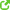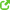# Chemical formulas

This is a very basic question. When I see a chemical formula, like for example combustion of methane: $CH_4+2O_2->CO_2+2H_20$ and I compute the standard enthalpy of reaction $H_r$, do I have always to think about this value $H$ and about the chemical reaction as describing a "certain amount", big from the microscopic point of view, of methane reacting with a certain amount of oxygen? Told another way, is it possible to interpret the chemical formula as describing a reaction between a single atom of methane with a singole molecule of oxygen (with no other methane molecules or oxygen present?)
My answer would be no, because we have always to talk about substances in thermodynamic equilibrium but I wanted to ask the opinion of somebody more expert than me.

This is a very basic question. When I see a chemical formula, like for example combustion of methane: $CH_4+2O_2->CO_2+2H_20$ and I compute the standard enthalpy of reaction $H_r$, do I have always to think about this value $H$ and about the chemical reaction as describing a "certain amount", big from the microscopic point of view